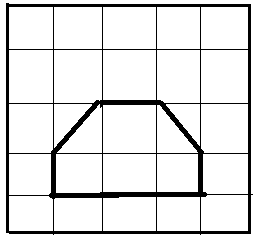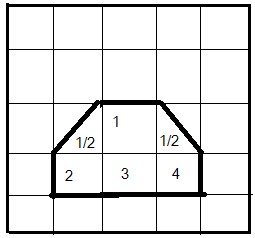QuestionAnswers

# If the area of one square is 1 square unit then find the area of the following figure by counting squares.Hint- A square is a polygon having four sides and four vertices such that each of the sides is equal and each of the vertices is at 90 degrees with each other. Also, the sum of the interior angles of the square equals 360 degrees.
In the question, it is asking for the area enclosed by the broad lines which encloses some full squares and some half-squares. So, first, we need to determine the total number of full squares and the total number of half squares, and to get the result, add them up to get the total number of full squares such that the area of a square is 10 square units.

Complete step by step solution:
Here, the area enclosed by the broad line is to be determined in which 4 complete squares are enclosed along with 2 half-squares such that in total of 5 complete squares are enclosed.As the area enclosed by one square is 1 square unit then, the area enclosed by 5 squares will be:
$A = 5 \times 1 \\ = 5{\text{ square units}} \\$

Hence, the area enclosed by the broad line is 5 square units.

Note: Alternatively, we can also proceed with the fact that if the area of a full-square is 1 square unit the, the area of the half-square is 0.5 square units and then 4 square units (for 4 complete squares) with two 0.5 square units (for two-half squares) to get the area enclosed by the broad lines.